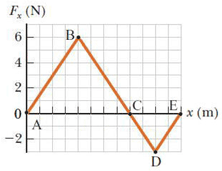# Problem: The force acting on a particle varies as in the figure below. (The x axis is marked in increments of 1.50 m.)    Find the work done by the force as the particle moves across the following distances.     from x = 0 m to x = 18.0 m

###### FREE Expert Solution

The work done is the sum of the two areas:

94% (325 ratings)###### Problem Details

The force acting on a particle varies as in the figure below. (The x axis is marked in increments of 1.50 m.)Find the work done by the force as the particle moves across the following distances.

from x = 0 m to x = 18.0 m

Frequently Asked Questions

What scientific concept do you need to know in order to solve this problem?

Our tutors have indicated that to solve this problem you will need to apply the More Work-Energy Problems concept. You can view video lessons to learn More Work-Energy Problems. Or if you need more More Work-Energy Problems practice, you can also practice More Work-Energy Problems practice problems.

What professor is this problem relevant for?

Based on our data, we think this problem is relevant for Professor Pagnotta's class at COFC.